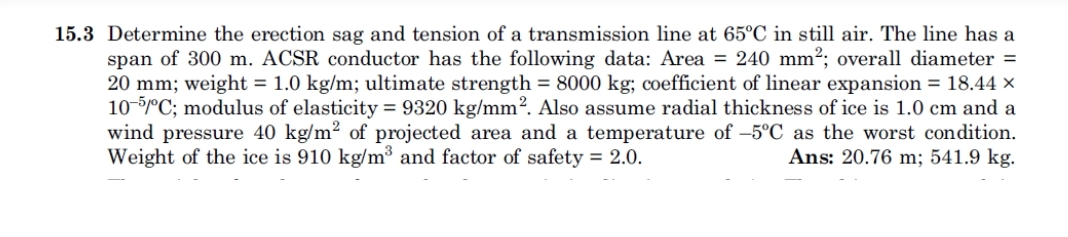# 15.3 Determine the erection sag and tension of a transmission line at 65°C in still air. The line has a span of 300 m. ACSR conductor has the following data: Area - 240 mm2; overall diameter - 20 mm , weight = 1.0 kg/m; ultimate strength = 8000 kg; coefficient of linear expansion = 18.44 x 10-5mC; modulus of elasticity = 9320 kg/mm2. Also assume radial thickness of ice is 1.0 cm and a wind pressure 40 kg/m2 of projected area and a temperature of -5°C as the worst condition. Ans: 20.76 m; 541.9 kg. Weight of the ice is 910 kg/m3 and factor of safety - 2.0.

Questionhelp_outlineImage Transcriptionclose15.3 Determine the erection sag and tension of a transmission line at 65°C in still air. The line has a span of 300 m. ACSR conductor has the following data: Area - 240 mm2; overall diameter - 20 mm , weight = 1.0 kg/m; ultimate strength = 8000 kg; coefficient of linear expansion = 18.44 x 10-5mC; modulus of elasticity = 9320 kg/mm2. Also assume radial thickness of ice is 1.0 cm and a wind pressure 40 kg/m2 of projected area and a temperature of -5°C as the worst condition. Ans: 20.76 m; 541.9 kg. Weight of the ice is 910 kg/m3 and factor of safety - 2.0. fullscreen

### Want to see the step-by-step answer?